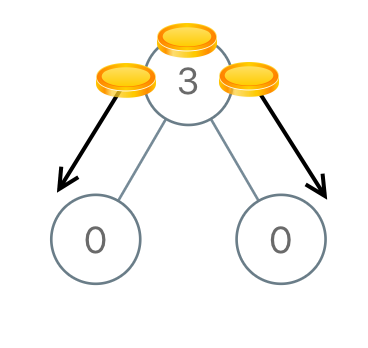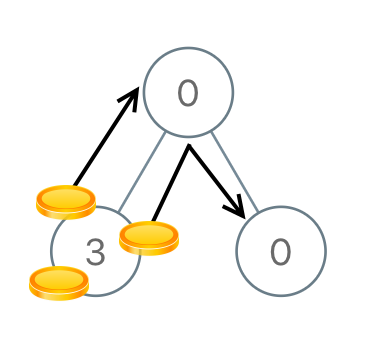## Algorithm

Problem Name: 979. Distribute Coins in Binary Tree

You are given the `root` of a binary tree with `n` nodes where each `node` in the tree has `node.val` coins. There are `n` coins in total throughout the whole tree.

In one move, we may choose two adjacent nodes and move one coin from one node to another. A move may be from parent to child, or from child to parent.

Return the minimum number of moves required to make every node have exactly one coin.

Example 1:```Input: root = [3,0,0]
Output: 2
Explanation: From the root of the tree, we move one coin to its left child, and one coin to its right child.
```

Example 2:```Input: root = [0,3,0]
Output: 3
Explanation: From the left child of the root, we move two coins to the root [taking two moves]. Then, we move one coin from the root of the tree to the right child.
```

Constraints:

• The number of nodes in the tree is `n`.
• `1 <= n <= 100`
• `0 <= Node.val <= n`
• The sum of all `Node.val` is `n`.

## Code Examples

### #1 Code Example with Java Programming

```Code - Java Programming```

``````
class Solution {
int ans;
public int distributeCoins(TreeNode root) {
ans = 0;
dfs(root);
return ans;
}

private int dfs(TreeNode root) {
if (root == null) {
return 0;
}
int l = dfs(root.left);
int r = dfs(root.right);
ans += Math.abs(l) + Math.abs(r);
return root.val + l + r - 1;
}
}
``````
Copy The Code &

Input

cmd
root = [3,0,0]

Output

cmd
2

### #2 Code Example with Javascript Programming

```Code - Javascript Programming```

``````
const distributeCoins = function(root) {
let res = 0
helper(root)
return res

function helper(node) {
if(node == null) return 0
const left = helper(node.left)
const right = helper(node.right)
res += Math.abs(left) + Math.abs(right)
return node.val + left + right - 1
}
};
``````
Copy The Code &

Input

cmd
root = [3,0,0]

Output

cmd
2

### #3 Code Example with Python Programming

```Code - Python Programming```

``````
class Solution:
def distributeCoins(self, root):
self.ans = 0

def dfs(node):
if not node: return 0
L, R = dfs(node.left), dfs(node.right)
self.ans += abs(L) + abs(R)
return node.val + L + R - 1

dfs(root)
return self.ans
``````
Copy The Code &

Input

cmd
root = [0,3,0]

Output

cmd
3

### #4 Code Example with C# Programming

```Code - C# Programming```

``````
using System;

namespace LeetCode
{
public class _0979_DistributeCoinsInBinaryTree
{
private int result = 0;

public int DistributeCoins(TreeNode root)
{
result = 0;
DistributeCoinsCore(root);
return result;
}

public int DistributeCoinsCore(TreeNode node)
{
if (node == null) return 0;
var left = DistributeCoinsCore(node.left);
var right = DistributeCoinsCore(node.right);
result += Math.Abs(left) + Math.Abs(right);
return left + right + node.val - 1;
}
}
}
``````
Copy The Code &

Input

cmd
root = [0,3,0]

Output

cmd
3